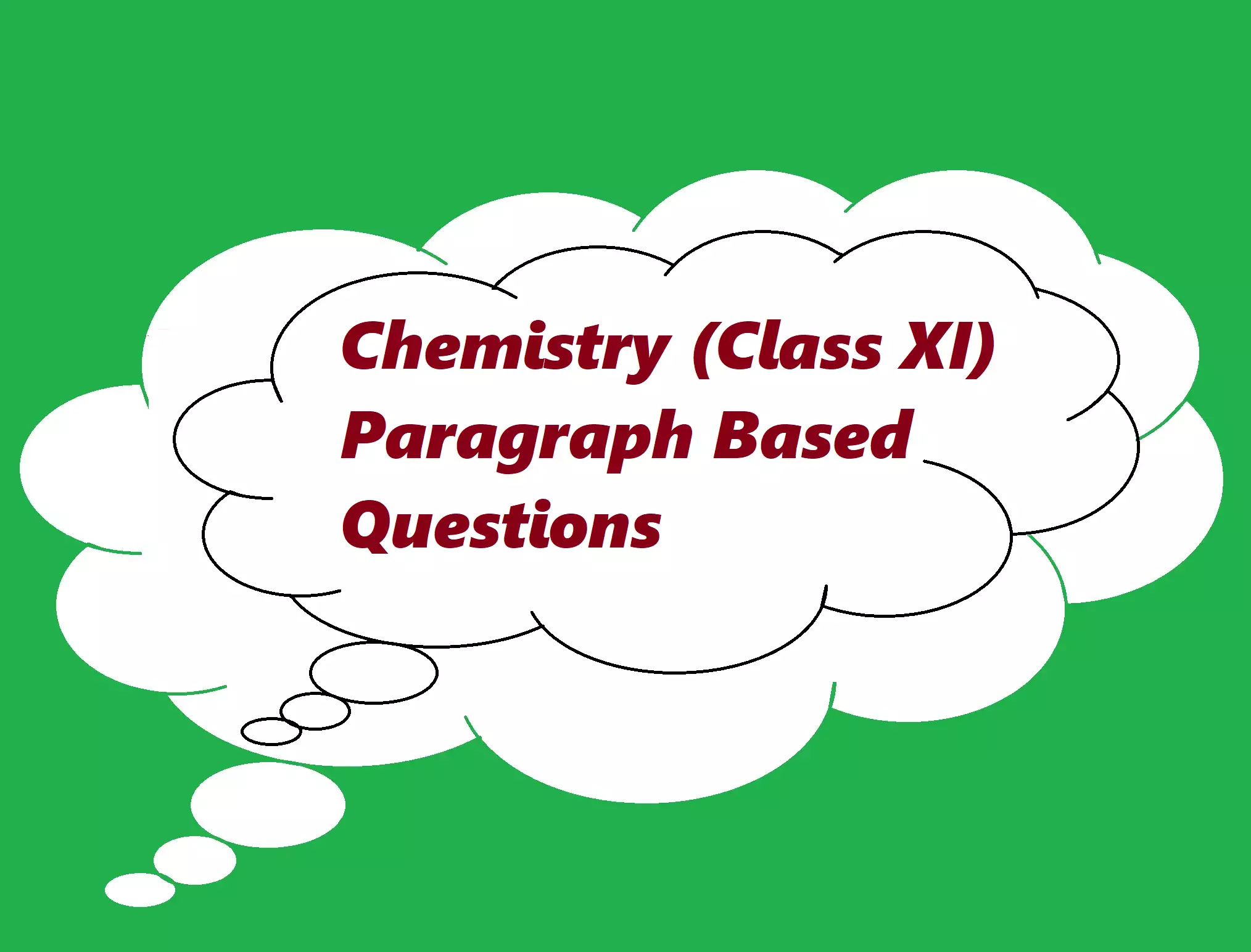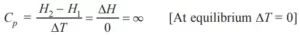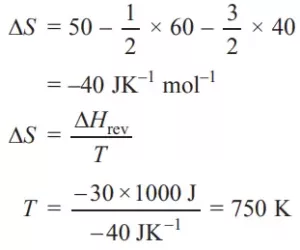# Chemistry (Class XI)Paragraph Based QuestionsChemistry (Class XI)Paragraph Based Questions

Chemistry (Class XI)Paragraph Based Questions

Boron is an element of Group 13. It is a metalloid. Borax, orthoboric acid and diborane are important compounds of boron. Borax is used to prepare heat-resistant pyrex glass. It is also used for cleaning metals like gold. It is also used in the borax bead test to detect colored salts in the lab. Orthoboric acid is a weak acid used as an antiseptic in eye lotion. It is solid having extensive H-bonding. It acts as Lewis acid. Diborane is a colorless, highly toxic gas. It catches fire spontaneously upon exposure to air. It burns in oxygen releasing an enormous amount of energy.
Que (i) Give one use of NaBH4.
Ans (i). Sodium Borohydride(NaBH4) reduces aldehydes and ketones to give the related alcohols.
Que (ii) Why is boric acid considered as weak Lewis acid?
Ans (ii). Because it is not able to release H+ ions on its own. It receives OH from the water molecule to complete its octet and in turn, releases H+ ions, hence acts as weak Lewis acid.
B(OH)3 + 2H2O → [B(OH)4] + H3O+
Que (iii) What happens when boric acid is heated strongly?
Ans (iii). B2O3 is formed as the following reaction.
H3BO3       →      HBO2             →       B2O3
Boric acid        Metaboric acid        Boron trioxide
Que (iv) What happens when sodium hydride reacts with B2H6?
Ans (iv). Sodium borohydride is formed.
2NaH + B2H6  →  2NaBH4
Sodium                 Sodium borohydride
hydride
Que (v) What happens when sodium borohydride is reacted with I2?
Ans (v). B2H6, NaI, and H2 are formed.
2NaBH4 + I2 → 2NaI + B2H6 + H2

Chemistry (Class XI)Paragraph Based Questions

The first element of each group, lithium in the first group and beryllium in the second group differs from the rest of the elements but have similar properties to diagonally situated elements. Lithium resembles Mg and Be resembles Aluminium. Boron resembles with Silicon. The compounds of alkali metals are predominantly ionic. The oxides and hydroxides are soluble in water forming strong alkalis. Important compounds of sodium include sodium carbonate, sodium chloride, sodium hydroxide, and NaHCO3. Sodium hydroxide is manufactured by the Castner-Kellner process and sodium carbonate by the Solvay process. The oxides and hydroxides of group 2 elements are less basic than group 1, oxides, and hydroxides. CaO, Ca(OH)2, CaSO4.1/2 H2O, CaCO3, and cement are industrially important compounds. Portland cement is an important constructional material. It is manufactured by heating limestone and clay in a rotary kiln. The clinker obtained is mixed with 2 to 3% gypsum. Na+, K+, Mg2+, and Ca2+ are found in large amounts in biological fluids. These ions perform important biological functions such as maintenance of ion balance and nerve impulse conductions.
Que (i) What is the cause of the diagonal relationship?
Ans (i). It is due to similar ionic radii.
Que (ii) Why does the first element of each group differ from the rest of the elements?
Ans (ii). It is due to its exceptionally small size, high polarizing power (charge/radius ratio).
Que (iii). Name the product formed at anode and cathode on electrolysis of brine solution.
Ans (iii). H2 is formed at the cathode, Cl2 is formed at the anode.
Que (iv) Why is BeSO4 soluble in water but BaSO4 is not?
Ans (iv). It is because Be2+ has higher hydration energy than Ba2+.
Que (v). Why is gypsum is added to cement?
Ans (v). It is done to delay the setting time of cement.
Que (vi). What is the role of Ca2+ in our body?
Ans (vi). It is present in bones and teeth. It plays important role in neuromuscular functions, interneuron transmission, and blood coagulation.
Que (vii). What is the role of Na+ in blood plasma?
Ans (vii). Na+ ions are present outside the cell, are located in blood plasma and fluids which surround the cells, and these ions help in the transmission of nerve signals.

Chemistry (Class XI)Paragraph Based Questions

We can measure the transfer of heat from one system to another which causes a change in temperature. The magnitude of change in temperature depends upon the heat capacity of the substance. The enthalpy change of reaction remains the same irrespective of the number of steps is Hess’s law. It helps to calculate the enthalpy of formation, combustion, and other enthalpy changes. Enthalpy change can also be calculated by using bond enthalpies. The first law gives the law of conservation of energy but does not give the direction of the reaction. The second law states, the entropy of the universe is continuously increasing due to spontaneous processes taking place in it. ΔH and ΔS (entropy change) cannot decide the spontaneity of the process. We need ΔG (free energy change) which is –ve for spontaneous, +ve for non-spontaneous. ΔG = 0 for the process in equilibrium. ΔG is related to the equilibrium constant. If ΔG = –ve, ‘K’ is +ve and vice versa. The third law of thermodynamics states the entropy of a perfectly crystalline substance is zero at zero kelvin.
Que (i). We can determine ΔHlattice with the help of the cycle. Name the cycle.
Ans (i). The cycle is the Born-Haber cycle.
Que (ii). How can we calculate the enthalpy of the solution?
Ans (ii). ΔH°solution = ΔH°lattice + ΔH°hydration.
Que (iii) What is the molar heat capacity of water in equilibrium with ice at constant pressure?
Ans (iii). ∞ (infinity).Que (iv).ΔHf° of O3, CaO, NH3, and HI are +142.2, – 643.9, –46, +25.95 kJ mol–1. Arrange these in increasing order of stability.
Ans (iv). O3 < HI < NH3 < CaO
Que (v). Standard entropy of X2, Y2, and XY3 are 60, 40, and 50 JK–1 mol–1 respectively. For the reaction 1/2X2 + 2/3 Y2 → XY3, ΔH = –30 kJ to be at what temperature, the process will be at equilibrium.
Ans (v).Que (vi). What are the signs of ΔH and ΔS for the process to be always spontaneous?
Ans (vi). ΔH = –ve, ΔS = +ve.
Que (vii). Give the mathematical expression for the second law of thermodynamics.
Ans (vii). ΔStotal > 0

Chemistry (Class XI)Paragraph Based Questions

In ionic compounds, there is an electrostatic force between oppositely charged ions. Covalent bonds are present in polar as well as non-polar molecules. Polar molecules have a dipole-dipole attraction. Polar molecules with non-polar molecules have dipole-induced dipole attraction, non-polar molecules have induced dipole-induced dipole, dispersion forces (London forces). H-bonds are present in some polar molecules. Ion-dipole attraction exists between ions and polar molecules intermolecular forces are negligible in a gaseous state with large intermolecular space. The interdependence of some observable properties pressure, volume, temperature, and mass lead to different gas laws, Boyle’s law, Charle’s law, Gay-Lussac’s law, Avogadro’s law, and Dalton’s law of partial pressure. The ideal gas equation PV = nRT is followed by ideal gases. At high pressure and low temperature, intermolecular forces start operating strongly and molecules come close to each other. They show positive and negative deviation from ideal gas behavior and follow van der Waals’ equation. Gases can be liquefied at low temperatures and high pressure. Liquids have more intermolecular forces of attraction. Some properties of liquids, e.g., surface tension and viscosity are due to strong attractive forces. Molecules of gases are in constant random motion. Their velocity can be expressed as average velocity, root mean square velocity, and most portable velocity.
Que (i). Under what conditions real gases show ideal gas behavior?
Ans (i). Low pressure and high temperature.
Que (ii). What type of forces of attraction exists between NO3 and water?
Ans (ii). Ion-dipole attraction.
Que (iii). How are different types of velocities related to molar mass?
Ans (iii). Velocities are inversely proportional to the square root of molar mass.
Que (iv). A gas at 350 K and 15 bar have a molar volume of 20% less than that for an ideal gas under the same conditions. What is the value of ‘Z’?
Ans (iv). Z = VReal/VIdeal = 0.8
Que (v). Why is HF liquid, HCl, HBr, HI are gases?
Ans (v). HF molecules are associated with intermolecular H-bonding.
Que (vi). How is the pressure of dry gas calculated?
Ans (vi). Pdry gas = Pmoist gas – Aqueous tension (Vapour pressure of water vapours).
Que (vii). What is Boyle’s temperature?
Ans (vii). It is the temperature at which most of the gases show ideal gas behavior over a wide range of pressure.

Chemistry Solved Question Paper Class XI
https://www.sciencemotive.com/class11/chemistry-solved-question-paper-class-xi/

Chemistry (Class XI)Paragraph Based Questions

Chemistry (Class XI)Paragraph Based Questions